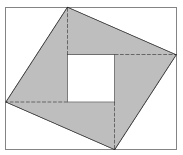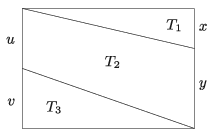Mathematical and Physical Journal
for High Schools
Issued by the MATFUND Foundation
 Already signed up? New to KöMaL?

# KöMaL Problems in Mathematics, February 2020

Show/hide problems of signs:## Problems with sign 'K'

Deadline expired on March 10, 2020.

K. 649. A fast train and a slow train of the same length are travelling on two parallel tracks, in opposite directions. The tracks both pass through a tunnel. The fronts of the two trains arrive at the two entrances of the tunnel simultaneously. It takes 3 seconds for the total length of the fast train to become covered by the tunnel, and it takes 6 seconds for the slow train. The trains meet inside the tunnel 18 seconds after reaching the tunnel. How long do they take to pass each other? At what time after meeting will the full length of the individual trains emerge from the tunnel?

(6 pont)

solution (in Hungarian), statistics

K. 650. The side of the small square in the figure is 3 cm, the sides of the large rectangle have integer lengths, one being 2 cm longer than the other. The sides of the rectangle and the square are parallel, their centres coincide. The shaded region was made by extending the sides of the small square in one direction, and connecting the points where the sides of the large rectangle were reached. Is it possible for the area of the shaded region to be an even number (of cm$\displaystyle {}^2$)?(6 pont)

solution (in Hungarian), statistics

K. 651. For the areas marked in the figure, $\displaystyle T_1 : T_2 : T_3 = 2:7:3$. What are the ratios of the lengths $\displaystyle x$ to $\displaystyle y$, and $\displaystyle u$ to $\displaystyle v$?(6 pont)

solution (in Hungarian), statistics

K. 652. A box contains yellow, blue and red balls, 10 of each colour. In how many different ways is it possible to divide the balls into a group of 10 and a group of 20 such that each group should contain at least one of each colour? (Balls of the same colour cannot be distinguished.)

(6 pont)

solution (in Hungarian), statistics

K. 653. We know that $\displaystyle \sqrt{a\sqrt{a\sqrt{a}}}=b$ and $\displaystyle a, b > 1$ are integers. Find the minimum value of $\displaystyle a+b$.

(6 pont)

solution (in Hungarian), statistics## Problems with sign 'C'

Deadline expired on March 10, 2020.

C. 1588. Let $\displaystyle E$ and $\displaystyle F$ be the points lying closer to vertex $\displaystyle A$ which divide the sides $\displaystyle AB$ and $\displaystyle AD$ of a quadrilateral $\displaystyle ABCD$, respectively, in a $\displaystyle 1:2$ ratio. Let $\displaystyle G$ be the point lying closer to $\displaystyle B$ which divides side $\displaystyle BC$ in a $\displaystyle 1:2$ ratio. Reflect point $\displaystyle G$ in the point $\displaystyle E$, and reflect its image in the point $\displaystyle F$. Prove that the final image lies on a side of the quadrilateral. Which side is it, and in what ratio is it divided by the final image?

(5 pont)

solution (in Hungarian), statistics

C. 1589. Solve the following equation over the set of real numbers:

$\displaystyle {(y^2+y-x-1)}^2+\left(x+\frac1x \right)^{2}=4.$

Proposed by B. Bíró, Eger

(5 pont)

solution (in Hungarian), statistics

C. 1590. Find the positive integer solutions of the equation

$\displaystyle {(a+1)}^4\cdot {(b+1)}^4\cdot {(c+1)}^4=(40a+1)\cdot(40b+1)\cdot(40c+1).$

(5 pont)

solution (in Hungarian), statistics

C. 1591. The coordinates of a ship are $\displaystyle x=2$, $\displaystyle y=0$. The shoreline is given by the curve of equation $\displaystyle y=\sqrt{2x+1}$. At what angle should the ship deviate from the direction due north in order to reach the closest point of the shore in a straight line? (Assume that the $\displaystyle x$-axis points towards the east.)

(5 pont)

solution (in Hungarian), statistics

C. 1592. In England, a man lost his wedding ring, and set out to search for it with the help of a friend and a metal detector. They did not find the ring, but they did find some gold coins from the time of King Henry VIII, delivering $\displaystyle 100\,000$ pounds to the two friends. The one-pound coins were preserved in very good condition, and during the 500 years elapsed, their average annual increase in value was between 1.42% and 1.43%. How many coins may they have found?

(5 pont)

solution (in Hungarian), statistics

C. 1593. Two sides of a triangle are 3 cm and 4 cm long. What is the angle enclosed by the sides if the medians drawn from the opposite vertices are perpendicular to each other?

(5 pont)

solution (in Hungarian), statistics

C. 1594. The first row of seats in an auditorium consists of 24 seats. 20 seats are already taken. What is the probability that there are 2 adjacent vacant seats?

(5 pont)

solution (in Hungarian), statistics## Problems with sign 'B'

Deadline expired on March 10, 2020.

B. 5078. Define a sequence $\displaystyle a_1,a_2,\ldots$ by the following recurrence relation:

$\displaystyle a_1=1, \quad a_n=\frac{n+1}{n-1}(a_1+a_2+\dots+a_{n-1}),\quad\text{for $$\displaystyle n>1$.}$$

Determine the value of $\displaystyle a_{2020}$.

(4 pont)

solution (in Hungarian), statistics

B. 5079. Solve the equation

$\displaystyle \log_2 \log_3 x+\log_3\log_2 x=\log_2\frac{6}{\log_2 3}$

over the set of real numbers.

Proposed by B. Bíró, Eger

(3 pont)

solution (in Hungarian), statistics

B. 5080. Let $\displaystyle D$ denote the midpoint of the base $\displaystyle AB$ in an isosceles triangle $\displaystyle ABC$. Let $\displaystyle H$ be the point lying closer to $\displaystyle C$ that divides the leg $\displaystyle AC$ in a $\displaystyle 1:2$ ratio. The circle $\displaystyle BCH$ intersects line $\displaystyle CD$ at the points $\displaystyle C$ and $\displaystyle X$. Show that $\displaystyle CX=\frac 43r$, where $\displaystyle r$ is the radius of the circle $\displaystyle ABC$.

(4 pont)

solution (in Hungarian), statistics

B. 5081. In a triangle, the medians drawn to sides $\displaystyle a$ and $\displaystyle b$ are perpendicular. Prove that $\displaystyle \frac 12<\frac ab<2$.

(3 pont)

solution (in Hungarian), statistics

B. 5082. Prove that the geometric mean, the arithmetic mean, and the quadratic mean of the altitudes in any triangle is not greater than the geometric mean, the arithmetic mean, and the quadratic mean of the radii of the escribed circles, respectively.

(5 pont)

solution (in Hungarian), statistics

B. 5083. Is there a polynomial $\displaystyle p(x)$ of degree 100 with real coefficients for which the polynomial $\displaystyle p\big(p(x)\big)$ has 10000 distinct real roots?

(5 pont)

solution (in Hungarian), statistics

B. 5084. Let $\displaystyle n$ be a positive integer, and let $\displaystyle \mathcal{S}$ denote the set of $\displaystyle 0-1-2$ sequences of length $\displaystyle n$. Determine which sets $\displaystyle \emptyset\ne A\subseteq\mathcal{S}$ have the following property: no matter how a vector

$\displaystyle (c_1,c_2,\ldots,c_n)\in \mathcal{S}\setminus\big\{(0,0,\dots,0)\big\}$

is selected, the probabilities that the sum of products $\displaystyle c_1a_1+c_2a_2+\dots+c_na_n$ formed with a randomly chosen element $\displaystyle (a_1,a_2,\ldots,a_n)$ of set $\displaystyle A$ will leave a remainder of $\displaystyle 0$, $\displaystyle 1$, or $\displaystyle 2$ are $\displaystyle 1/3$ each.

Based on a problem of Kürschák competition

(6 pont)

solution (in Hungarian), statistics

B. 5085. Show that a regular heptagon can be dissected into a finite number of symmetrical trapezoids, all similar to each other.

Proposed by M. Laczkovich, Budapest

(6 pont)

solution (in Hungarian), statistics## Problems with sign 'A'

Deadline expired on March 10, 2020.

A. 769. Find all triples $\displaystyle (a,b,c)$ of distinct positive integers so that there exists a subset $\displaystyle S$ of the positive integers for which for all positive integers $\displaystyle n$ exactly one element of the triple $\displaystyle (an,bn,cn)$ is in $\displaystyle S$.

Proposed by Carl Schildkraut, Massachussets Institute of Technology

(7 pont)

statistics

A. 770. Find all positive integers $\displaystyle n$ such that $\displaystyle n!$ can be written as the product of two Fibonacci numbers.

(7 pont)

solution (in Hungarian), statistics

A. 771. Let $\displaystyle \omega$ denote the incircle of triangle $\displaystyle ABC$, which is tangent to side $\displaystyle BC$ at point $\displaystyle D$. Let $\displaystyle G$ denote the second intersection of line $\displaystyle AD$ and circle $\displaystyle \omega$. The tangent to $\displaystyle \omega$ at point $\displaystyle G$ intersects sides $\displaystyle AB$ and $\displaystyle AC$ at points $\displaystyle E$ and $\displaystyle F$. The circumscribed circle of $\displaystyle DEF$ intersects $\displaystyle \omega$ at points $\displaystyle D$ and $\displaystyle M$. The circumscribed circle of $\displaystyle BCG$ intersects $\displaystyle \omega$ at point $\displaystyle G$ and $\displaystyle N$. Prove that lines $\displaystyle AD$ and $\displaystyle MN$ are parallel.

Proposed by Ágoston Győrffy, Remeteszőlős

(7 pont)

solution (in Hungarian), statistics